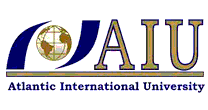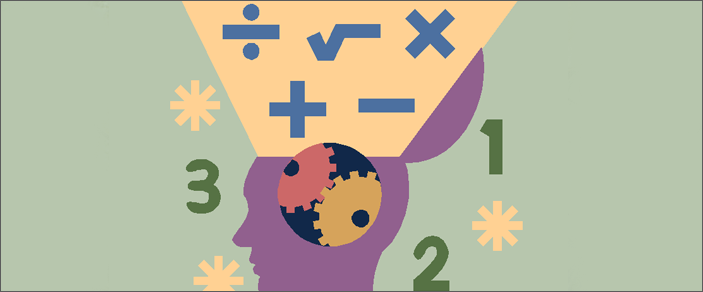﻿ Fundamentals of Math - Open Courses by Atlantic International University - AIU#### Fundamentals of Math CourseFundamentals of Math Course

The following course in Fundamentals of Math is provided in its entirety by Atlantic International University's "Open Access Initiative " which strives to make knowledge and education readily available to those seeking advancement regardless of their socio-economic situation, location or other previously limiting factors. The University's Open Courses are free and do not require any purchase or registration, they are open to the public.

Objectives for Fundamentals of Math:

• Find the perimeter, and area of squares, parallelograms, triangles, trapezoids and circles; volume and surface area, relations between angle measures, congruent and similar triangles, and properties of parallelograms.
• Add, subtract, multiply and divide real numbers and manipulate certain expressions.
• Solve problems using equations and inequalities.
• Add, subtract, multiply and factor polynomials.
• Add, subtract, multiply and divide, and simplify rational expressions

Description for Fundamentals of Math:

• Find the perimeter, and area of squares, parallelograms, triangles, trapezoids and circles; volume and surface area, relations between angle measures, congruent and similar triangles, and properties of parallelograms.
• Add, subtract, multiply and divide real numbers and manipulate certain expressions.
• Solve problems using equations and inequalities.
• Add, subtract, multiply and factor polynomials.
• Add, subtract, multiply and divide, and simplify rational expressions

Introduction: Fundamentals of Math (Open)
This brief Video Introduces the professor and topics to be covered in the course

Course Content, Lessons, and Tests: Fundamentals of Math (Open)
The lessons and topics for this course are provided in audio and video format. A test will follow the conclusion of each lesson to verify the concepts covered have been learned.

Review of Topics and Lessons: Fundamentals of Math (Open)
A outline of the topics covered in this course is provided in video format.

Conclusion: Fundamentals of Math (Open)